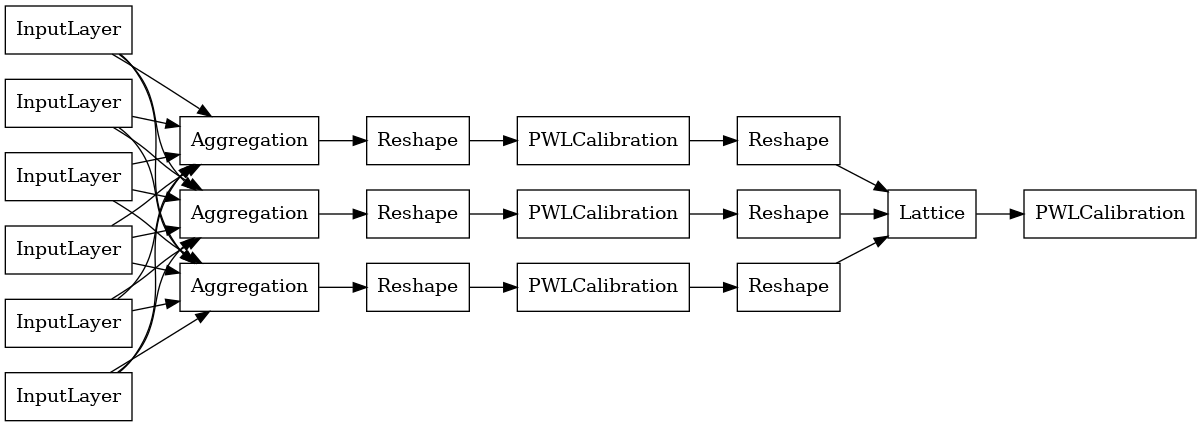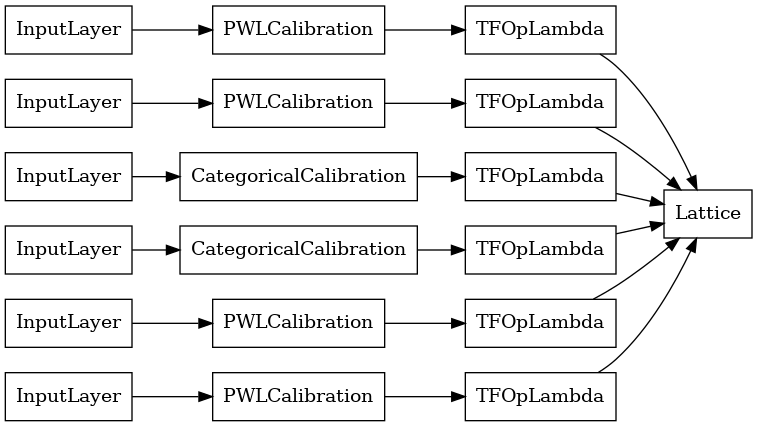Thanks for tuning in to Google I/O. View all sessions on demand

# TF Lattice Aggregate Function Models

## Overview

TFL Premade Aggregate Function Models are quick and easy ways to build TFL `tf.keras.model` instances for learning complex aggregation functions. This guide outlines the steps needed to construct a TFL Premade Aggregate Function Model and train/test it.

## Setup

Installing TF Lattice package:

````pip install tensorflow-lattice pydot`
```

Importing required packages:

``````import tensorflow as tf

import collections
import logging
import numpy as np
import pandas as pd
import sys
import tensorflow_lattice as tfl
logging.disable(sys.maxsize)
``````
```2022-12-16 12:35:04.017993: W tensorflow/compiler/xla/stream_executor/platform/default/dso_loader.cc:64] Could not load dynamic library 'libnvinfer.so.7'; dlerror: libnvinfer.so.7: cannot open shared object file: No such file or directory
2022-12-16 12:35:04.018100: W tensorflow/compiler/xla/stream_executor/platform/default/dso_loader.cc:64] Could not load dynamic library 'libnvinfer_plugin.so.7'; dlerror: libnvinfer_plugin.so.7: cannot open shared object file: No such file or directory
2022-12-16 12:35:04.018111: W tensorflow/compiler/tf2tensorrt/utils/py_utils.cc:38] TF-TRT Warning: Cannot dlopen some TensorRT libraries. If you would like to use Nvidia GPU with TensorRT, please make sure the missing libraries mentioned above are installed properly.
```

``````train_dataframe = pd.read_csv(
'https://raw.githubusercontent.com/wbakst/puzzles_data/master/train.csv')
``````
``````test_dataframe = pd.read_csv(
'https://raw.githubusercontent.com/wbakst/puzzles_data/master/test.csv')
``````

Extract and convert features and labels

``````# Features:
# - star_rating       rating out of 5 stars (1-5)
# - word_count        number of words in the review
# - is_amazon         1 = reviewed on amazon; 0 = reviewed on artifact website
# - includes_photo    if the review includes a photo of the puzzle
# - num_reviews       total number of reviews for this puzzle (we construct)
#
# This ordering of feature names will be the exact same order that we construct
# our model to expect.
feature_names = [
'num_reviews'
]
``````
``````def extract_features(dataframe, label_name):
# First we extract flattened features.
flattened_features = {
feature_name: dataframe[feature_name].values.astype(float)
for feature_name in feature_names[:-1]
}

# Construct mapping from puzzle name to feature.
star_rating = collections.defaultdict(list)
word_count = collections.defaultdict(list)
is_amazon = collections.defaultdict(list)
includes_photo = collections.defaultdict(list)
labels = {}

# Extract each review.
for i in range(len(dataframe)):
row = dataframe.iloc[i]
puzzle_name = row['puzzle_name']
star_rating[puzzle_name].append(float(row['star_rating']))
word_count[puzzle_name].append(float(row['word_count']))
is_amazon[puzzle_name].append(float(row['is_amazon']))
includes_photo[puzzle_name].append(float(row['includes_photo']))
labels[puzzle_name] = float(row[label_name])

# Organize data into list of list of features.
names = list(star_rating.keys())
star_rating = [star_rating[name] for name in names]
word_count = [word_count[name] for name in names]
is_amazon = [is_amazon[name] for name in names]
includes_photo = [includes_photo[name] for name in names]
num_reviews = [[len(ratings)] * len(ratings) for ratings in star_rating]
labels = [labels[name] for name in names]

# Flatten num_reviews
flattened_features['num_reviews'] = [len(reviews) for reviews in num_reviews]

# Convert data into ragged tensors.
star_rating = tf.ragged.constant(star_rating)
word_count = tf.ragged.constant(word_count)
is_amazon = tf.ragged.constant(is_amazon)
includes_photo = tf.ragged.constant(includes_photo)
num_reviews = tf.ragged.constant(num_reviews)
labels = tf.constant(labels)

# Now we can return our extracted data.
return (star_rating, word_count, is_amazon, includes_photo, num_helpful,
num_reviews), labels, flattened_features
``````
``````train_xs, train_ys, flattened_features = extract_features(train_dataframe, 'Sales12-18MonthsAgo')
test_xs, test_ys, _ = extract_features(test_dataframe, 'SalesLastSixMonths')
``````
```2022-12-16 12:35:05.723090: E tensorflow/compiler/xla/stream_executor/cuda/cuda_driver.cc:267] failed call to cuInit: CUDA_ERROR_NO_DEVICE: no CUDA-capable device is detected
```
``````# Let's define our label minimum and maximum.
min_label, max_label = float(np.min(train_ys)), float(np.max(train_ys))
min_label, max_label = float(np.min(train_ys)), float(np.max(train_ys))
``````

Setting the default values used for training in this guide:

``````LEARNING_RATE = 0.1
BATCH_SIZE = 128
NUM_EPOCHS = 500
MIDDLE_DIM = 3
MIDDLE_LATTICE_SIZE = 2
MIDDLE_KEYPOINTS = 16
OUTPUT_KEYPOINTS = 8
``````

## Feature Configs

Feature calibration and per-feature configurations are set using tfl.configs.FeatureConfig. Feature configurations include monotonicity constraints, per-feature regularization (see tfl.configs.RegularizerConfig), and lattice sizes for lattice models.

Note that we must fully specify the feature config for any feature that we want our model to recognize. Otherwise the model will have no way of knowing that such a feature exists. For aggregation models, these features will automaticaly be considered and properly handled as ragged.

### Compute Quantiles

Although the default setting for `pwl_calibration_input_keypoints` in `tfl.configs.FeatureConfig` is 'quantiles', for premade models we have to manually define the input keypoints. To do so, we first define our own helper function for computing quantiles.

``````def compute_quantiles(features,
num_keypoints=10,
clip_min=None,
clip_max=None,
missing_value=None):
# Clip min and max if desired.
if clip_min is not None:
features = np.maximum(features, clip_min)
features = np.append(features, clip_min)
if clip_max is not None:
features = np.minimum(features, clip_max)
features = np.append(features, clip_max)
# Make features unique.
unique_features = np.unique(features)
# Remove missing values if specified.
if missing_value is not None:
unique_features = np.delete(unique_features,
np.where(unique_features == missing_value))
# Compute and return quantiles over unique non-missing feature values.
return np.quantile(
unique_features,
np.linspace(0., 1., num=num_keypoints),
interpolation='nearest').astype(float)
``````

### Defining Our Feature Configs

Now that we can compute our quantiles, we define a feature config for each feature that we want our model to take as input.

``````# Feature configs are used to specify how each feature is calibrated and used.
feature_configs = [
tfl.configs.FeatureConfig(
name='star_rating',
lattice_size=2,
monotonicity='increasing',
pwl_calibration_num_keypoints=5,
pwl_calibration_input_keypoints=compute_quantiles(
flattened_features['star_rating'], num_keypoints=5),
),
tfl.configs.FeatureConfig(
name='word_count',
lattice_size=2,
monotonicity='increasing',
pwl_calibration_num_keypoints=5,
pwl_calibration_input_keypoints=compute_quantiles(
flattened_features['word_count'], num_keypoints=5),
),
tfl.configs.FeatureConfig(
name='is_amazon',
lattice_size=2,
num_buckets=2,
),
tfl.configs.FeatureConfig(
name='includes_photo',
lattice_size=2,
num_buckets=2,
),
tfl.configs.FeatureConfig(
lattice_size=2,
monotonicity='increasing',
pwl_calibration_num_keypoints=5,
pwl_calibration_input_keypoints=compute_quantiles(
# Larger num_helpful indicating more trust in star_rating.
reflects_trust_in=[
tfl.configs.TrustConfig(
feature_name="star_rating", trust_type="trapezoid"),
],
),
tfl.configs.FeatureConfig(
name='num_reviews',
lattice_size=2,
monotonicity='increasing',
pwl_calibration_num_keypoints=5,
pwl_calibration_input_keypoints=compute_quantiles(
flattened_features['num_reviews'], num_keypoints=5),
)
]
``````
```/tmpfs/tmp/ipykernel_35737/3271043037.py:20: DeprecationWarning: the `interpolation=` argument to quantile was renamed to `method=`, which has additional options.
Users of the modes 'nearest', 'lower', 'higher', or 'midpoint' are encouraged to review the method they used. (Deprecated NumPy 1.22)
return np.quantile(
```

## Aggregate Function Model

To construct a TFL premade model, first construct a model configuration from tfl.configs. An aggregate function model is constructed using the tfl.configs.AggregateFunctionConfig. It applies piecewise-linear and categorical calibration, followed by a lattice model on each dimension of the ragged input. It then applies an aggregation layer over the output for each dimension. This is then followed by an optional output piecewise-linear calibration.

``````# Model config defines the model structure for the aggregate function model.
aggregate_function_model_config = tfl.configs.AggregateFunctionConfig(
feature_configs=feature_configs,
middle_dimension=MIDDLE_DIM,
middle_lattice_size=MIDDLE_LATTICE_SIZE,
middle_calibration=True,
middle_calibration_num_keypoints=MIDDLE_KEYPOINTS,
middle_monotonicity='increasing',
output_min=min_label,
output_max=max_label,
output_calibration=True,
output_calibration_num_keypoints=OUTPUT_KEYPOINTS,
output_initialization=np.linspace(
min_label, max_label, num=OUTPUT_KEYPOINTS))
# An AggregateFunction premade model constructed from the given model config.
aggregate_function_model_config)
# Let's plot our model.
tf.keras.utils.plot_model(
aggregate_function_model, show_layer_names=False, rankdir='LR')
``````The output of each Aggregation layer is the averaged output of a calibrated lattice over the ragged inputs. Here is the model used inside the first Aggregation layer:

``````aggregation_layers = [
layer for layer in aggregate_function_model.layers
if isinstance(layer, tfl.layers.Aggregation)
]
tf.keras.utils.plot_model(
aggregation_layers.model, show_layer_names=False, rankdir='LR')
``````Now, as with any other tf.keras.Model, we compile and fit the model to our data.

``````aggregate_function_model.compile(
loss='mae',
aggregate_function_model.fit(
train_xs, train_ys, epochs=NUM_EPOCHS, batch_size=BATCH_SIZE, verbose=False)
``````
```<keras.callbacks.History at 0x7f76cfc1f4c0>
```

After training our model, we can evaluate it on our test set.

``````print('Test Set Evaluation...')
print(aggregate_function_model.evaluate(test_xs, test_ys))
``````
```Test Set Evaluation...
7/7 [==============================] - 2s 3ms/step - loss: 47.9940
47.99398422241211
```
[{ "type": "thumb-down", "id": "missingTheInformationINeed", "label":"Missing the information I need" },{ "type": "thumb-down", "id": "tooComplicatedTooManySteps", "label":"Too complicated / too many steps" },{ "type": "thumb-down", "id": "outOfDate", "label":"Out of date" },{ "type": "thumb-down", "id": "samplesCodeIssue", "label":"Samples / code issue" },{ "type": "thumb-down", "id": "otherDown", "label":"Other" }]
[{ "type": "thumb-up", "id": "easyToUnderstand", "label":"Easy to understand" },{ "type": "thumb-up", "id": "solvedMyProblem", "label":"Solved my problem" },{ "type": "thumb-up", "id": "otherUp", "label":"Other" }]